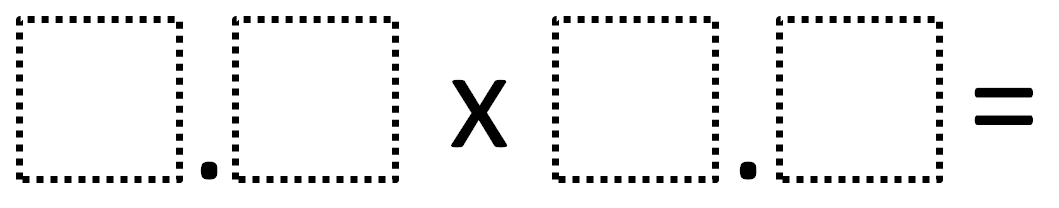# Decimal Product Close To 50

Directions: Using the digits 1 to 9 at most one time each, fill in the boxes so that the product is as close to 50 as possible.### Hint

What whole numbers would you multiply to make it as close to 50 as possible?
Closest is defined as the absolute value of the difference between the product and 50.

The closest products found are 50.02 (6.1 x 8.2) from a student in Shannon Andrews‘ class as well as 49.98 (9.8 x 5.1) from a student in Lauren Giordano’s class.

Source: Robert Kaplinsky

## Equivalent Expressions with Fractions

Directions: Using the digits 0 to 9 at most one time each and choosing either …

1.A student found 9.8 x 5.1 = 49.98

•Robert Kaplinsky

2.3.•Can only use each number once!

4.Is the problem as close to 50 without going over because I don’t see anything about that? If not, 8.2 x 6.1 is 50.02 and two hundredths away from 50 which is equal to 9.8 x 5.1 = 49.98 (distance wise).

•Never mind, I just saw it was added to the answers and I must have missed that!

5.9.8 x 5.1=49.98 or 6.1 x 8.2=50.02

6.